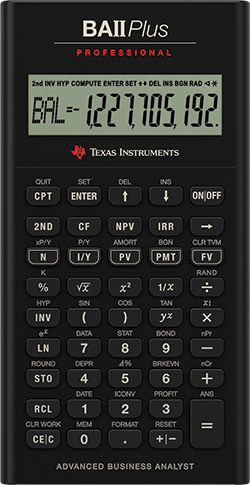The following are the authorised calculators for the Fellowship exams: (The suffix means any numbers or letters that follow the calculator's make and model)
Casio FX82 (with or without any suffix) Sharp EL531 (with or without any prefix or suffix)
Casio FX83 (with or without any suffix) Texas Instruments BA II Plus (with or without any suffix)
Casio FX85 (with or without any suffix) Texas Instruments TI-30 (with or without any suffix)
Hewlett Packard HP12c (with or without any suffix)

Jackie购买的是 TI-30XS 和 BA-II Plus Pro ，在中国精算师，英国精算师，北美精算师考试的时候都能用。

## 1.1 科学计算器的使用

CS1（对应旧体系的CT3 概率论与数理统计）科目中，在科学计算器中输入题中已有的数据即可直接求解一元线性回归模型中的参数 $$\alpha$$$$\beta$$，以及拟合优度 $$R^2$$。但此方法并不是对所有的题目都节省时间，而且从知识掌握的系统性角度考虑，不建议一开始就采用此方法做题，掌握好计算的原理才能以不变应万变。

## 1.2 金融计算器的使用• N 为时间（单位为年或期）（Number of Periods）

• I/Y为年利率或每期利率的100倍（effective interest rate in percentage

• PV 为现值（Present Value）

• PMT 为年金每年的付款额（Payment Amount）

• FV 为终值（积累值）（Future Value）

• CPT 是生成的意思（Compute）

• STO 存储计算数值；

• RCL 调用存储的值。

• 第一步，每次操作前需要清空 TVM。依次按 2ND $$\rightarrow$$ FV （即： CLR TVM
• 第二步，将其他几个已知量输入，再按 CPT $$\rightarrow$$ 未知量 即可得出结果。

### 1.2.1 单次支付的计算

• 输入时间：5 $$\rightarrow$$ N

• 输入年年利率：8 $$\rightarrow$$I/Y

• 输入现值：1234 $$\rightarrow$$ +/- $$\rightarrow$$ PV

• 计算积累值：CPT $$\rightarrow$$ FV

• 8 $$\rightarrow$$ I/Y

• 5 $$\rightarrow$$ N

• 1813.1508 $$\rightarrow$$ FV

• CPT $$\rightarrow$$ PV

• 8 $$\rightarrow$$ I/Y

• 1234 $$\rightarrow$$ +/- $$\rightarrow$$ PV

• 1813.1508 $$\rightarrow$$ FV

• CPT $$\rightarrow$$ N

• 5 $$\rightarrow$$ N

• 1234 $$\rightarrow$$ +/- $$\rightarrow$$ PV

• 1813.1508 $$\rightarrow$$ FV

• CPT $$\rightarrow$$ I/Y

• 6.25 $$\rightarrow$$ N

• 9.27 $$\rightarrow$$ +/- $$\rightarrow$$ I/Y

• 1000 $$\rightarrow$$ PV

• CPT $$\rightarrow$$ FV

• 6.25 $$\rightarrow$$ +/- $$\rightarrow$$ N

• 9.27 $$\rightarrow$$ +/- $$\rightarrow$$ I/Y

• 1000 $$\rightarrow$$ FV

• CPT $$\rightarrow$$ PV

### 1.2.2 年金的计算

#### 1.2.2.1 期末年金的计算

BA-II Plus 在默认状态时为计算期末年金（Annuity-immediate）的模式，屏幕上是没有 BGN 的，如果之前调整到了期初年金的模式，需要调回来，方法如下：

• 第一步，2ND $$\rightarrow$$ PMT

• 第二步，2ND $$\rightarrow$$ ENTER

• 第三步，2ND $$\rightarrow$$ CPT

• 清空 PV 里的数值：0 $$\rightarrow$$ PV

• 10 $$\rightarrow$$ +/- $$\rightarrow$$ PMT

• 7 $$\rightarrow$$ N

• 4 $$\rightarrow$$ I/Y

• CPT $$\rightarrow$$ PV

• 清空 TVM 。依次按 2ND $$\rightarrow$$ FV （即： CLR TVM

• 100 $$\rightarrow$$ +/- $$\rightarrow$$ PMT

• 9 $$\rightarrow$$ I/Y

• 912.85 $$\rightarrow$$ PV

• CPT $$\rightarrow$$ N

• 清空 FV 里的数值：0 $$\rightarrow$$ FV

• 2000 $$\rightarrow$$ +/- $$\rightarrow$$PMT

• 6 $$\rightarrow$$ N

• 7 $$\rightarrow$$ I/Y

• CPT $$\rightarrow$$ FV

• 首先按照上一道题的步骤计算出 $$PMT \times \sx{\angl{6}}$$, 屏幕上会显示计算结果 8992.614457

• 把上述数值存储在1这个单元里：STO $$\rightarrow$$ 1

• 把1单元里存储的数值调出来并设置为 PV：RCL $$\rightarrow$$ 1 $$\rightarrow$$ PV

• 4 $$\rightarrow$$N

• 7 $$\rightarrow$$ I/Y

• 清空 FV 里的数值：0 $$\rightarrow$$ FV

• CPT $$\rightarrow$$ PMT

#### 1.2.2.2 期初年金的计算

• 第一步，2ND $$\rightarrow$$ PMT

• 第二步，2ND $$\rightarrow$$ ENTER

• 第三步，2ND $$\rightarrow$$CPT

• 100 $$\rightarrow$$ +/- $$\rightarrow$$ PMT

• 60 $$\rightarrow$$ N

• 4256.0024 $$\rightarrow$$PV

• CPT $$\rightarrow$$ I/Y

CM1利息理论 & 寿险精算一对一在线辅导招生中，详情点击推文了解 CM1利息理论&寿险精算 一对一在线辅导 开课了！